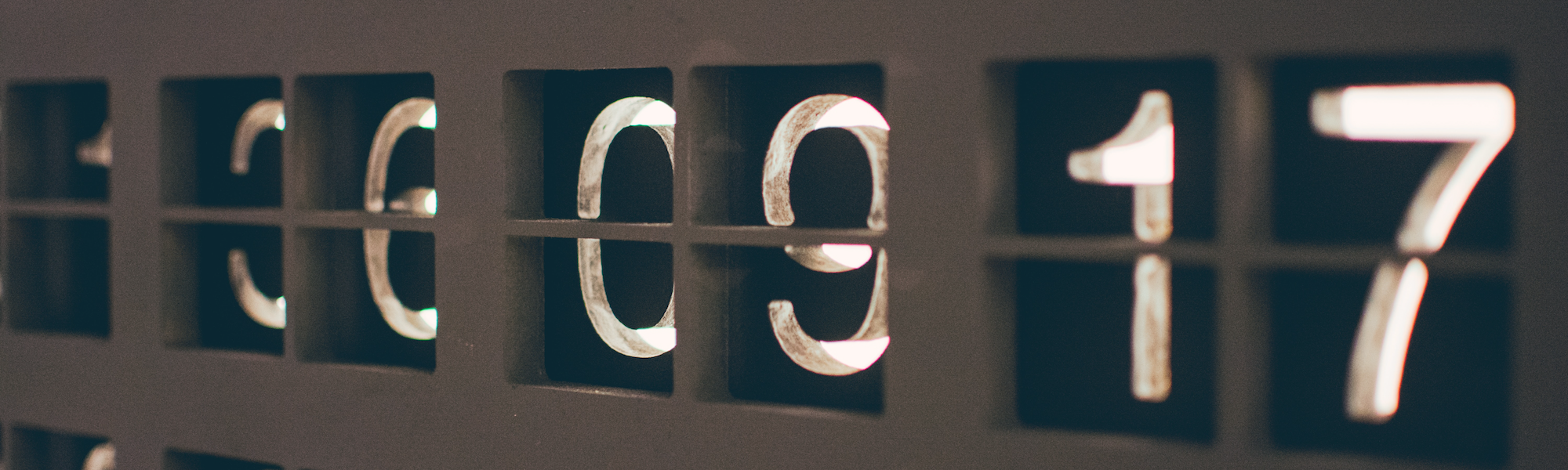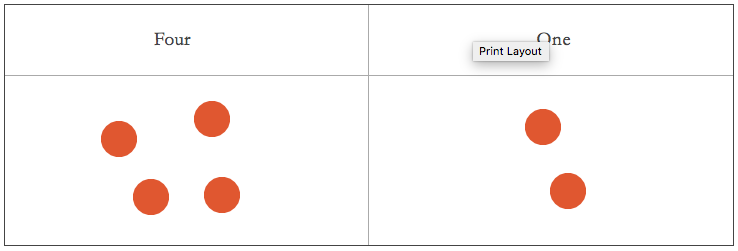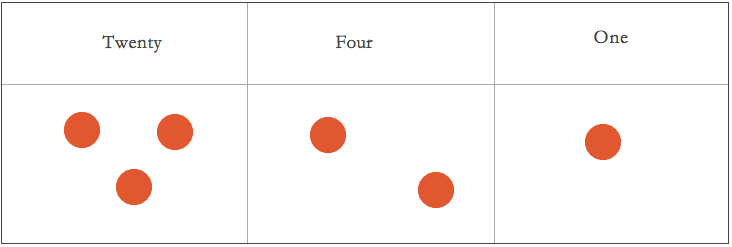Representing Positive Integers

Representation and Abstraction

We can gather a lot of information about a person's appearance by looking at a picture of their face. We may be able to identify the person in a crowd. We may also be able to figure out their skin-tone, or whether they have nose hair. We also know that if you punch the picture, the picture does not feel pain. Neither does the person who was represented by the picture (at least not physical pain!). So, the picture is not the person but a representation of the person.

A picture is also an abstraction. Abstraction here means that the picture leaves out certain details of the person's face. For instance, it is a two-dimensional representation of a three-dimensional object and so is bound to leave out specific information. The picture of a face may not tell you how broad or big the person's face is. But if someone points to a picture of a frog and of a person and asks you, 'which one of those is a person?' you will be able to make a clear choice. What you are saying here is not that the picture is a person but that the picture is a representation of a person. This is similar to what was mentioned above - 6, 32, and 9873 are numbers, but H, J, C, R, and X are not. The former set represents numbers in a particular numeral system. In contrast, the latter do not represent numbers in that numeral system.

Now, think of a child's black and white stick figure, which is meant to represent any person. Here, the child has left out many details such as skin tone, the shape of the ears, or whether the person has nose hair. But we can still understand that the child is representing a human. So, what the child is doing here is abstracting certain traits of humans they find commonly among humans they have seen. The stick figure is an abstract representation of any human.

You can similarly think of numbers. What do two horses and two tables have in common? What do seven frogs, seven planets, seven planetary systems, and seven buildings have in common? What is common is the concept of 'two' and 'seven.' We have abstracted away from specific objects such as planets, frogs, and tables. This allows us to answer questions such as 'are there more frogs in my house or more horses?' It is important to note that you can never actually see a number or touch it or feel it. You might say that you can see three cows or ten oranges. However, if I ask you to show me three or ten, you will not be able to do so. Showing me the numeral 3 or 10, or three fingers and ten fingers don't count. They are not numbers themselves but representations of numbers.

Various Representations of Numbers

There are many ways to represent a particular number. You might have been taught numbers through dots or lines when you were younger, with each dot or line representing the number one. Later, you were probably taught the Hindu Arabic number system. This is the number system where the numeral '6' represents the same thing as 'six.' The numeral '894' represents the same thing as 'eight hundred and ninety-four.' You might have even been taught how to use the Roman Numerals mentioned above. You might also have learned number names in various languages. Six, seis, ستة, छह, 六, זעקס, and έξι all represent the same number in English, Spanish, Arabic, Hindi, Mandarin, Yiddish, and Greek respectively. There is no 'correct' representation. We use one representation over others for reasons including social acceptance and ease of use.

Returning to Dots

Let us look again at the dots you used in primary school. Every number can be represented using these dots (we are only concerning ourselves with positive whole numbers). Dots/lines are the most obvious representations of numbers. The earliest found markings, which archaeologists consider to be numbers, are scratched lines. So, why did we create newer representations of numbers? Were dots and lines not good enough?

Look below. Without counting, how many dots do you see?Now, without counting, how many dots do you see?Most adults can answer correctly in the first case, while almost none can in the second. Counting more than thirty dots is a chore most people would rather avoid. Given the large numbers of objects we see around us, using dots to represent numbers is not a feasible option. So, what humans did when numbers got out of hand was to bunch things together and represent many single objects with a different symbol.Atomic and Complex Symbols

The picture above has the same number of dots as the previous one. However, you can quickly tell how many there are since the dots have been bunched into four units of four dots each, with two leftover. Just by bunching the dots together, we were able to make our lives easier. Here each dot is an 'atomic symbol,' and the collection of four dots is a 'complex symbol.' The value of a complex symbol is derived from the atomic symbols it is made of.

However, I still had to draw each dot (at least copy and paste them!), which took some effort. Now, how about if I do this:This time, rather than drawing eighteen objects, I only needed to draw six. As you can probably guess, each box represents four dots. What this gives us are two atomic symbols, the square, and the dot. The square's value is not given by its shape or its make-up, like was the case with the bunch of four dots. Instead, it has a value assigned to it. To take this further, you can use a triangle to represent, say, twenty dots, and so on.

However, as your numbers get bigger and bigger, the number of shapes will get larger and larger, and remembering them will become a chore. Another option is to change the color of the dots to represent a group of dots. Let the green dots be equivalent to the square in the picture above:Even here, the same problems of remembering colors will eventually hit you. In every representation, you are playing a game of give and take. In the case of just the dots, there was only one symbol/color to remember. However, to make our work easier, we had to increase the number of symbols/colors and hence our need to memorize. Representations almost always require some amount of compromise.

However, we can create more efficient methods of representation. There are many ways of simplifying representations. The first, which we have used above, involves changing the shape to represent a group of objects. Another way is through positioning. Notice that in the first, second, and fourth cases above, it does not matter what the shapes' position is. In the third it does, but only to make it easier to count. How do we use the relative position of symbols to simplify the representation? (Here, relative means in relation to each other)

Roman numerals are an example of a system that uses position to make it easier to count, but only to a limited extent. For all those who have not used Roman Numerals before, here is a sketch of how they work.

The Romans are Coming

Here are what some of the symbols translate to in Roman-Arabic numerals:

Roman Symbol Hindu-Arabic Translation
I 1
V 5
X 10
L 50
C 100
D 500
M 1000

Here are some numbers represented using the symbols above.

Roman Symbol Hindu-Arabic Translation
I 1
II 2
III 3
IV 4
V 5
VI 6
VII 8
IX 9
X 10

Initially, the numbers appear to work like our dots. The 'I' is equivalent to the dot. It is an 'atomic symbol.' All the symbols in the first table are 'atomic symbols.' 2 and 3 and 4 are represented by complex symbols, atomic symbols put together, and so are 6, 7, 8, and 9.

Clearly, Roman Numerals are using a similar approach to the one we used when we used squares as fours. If they used them in the same way, here would be how they could represent 444.This is not using position at all. However, Roman Numerals do not work exactly this way. In Roman Numerals, XI is 11, but IX is 9. So, putting a number to the left of another subtracts it away. However, this is not always the case. IIX is not 8, and IL is not 49. 8 is VIII while 49 is XLIX, and IIX and IL do not represent any number. To understand precisely how Roman Numerals work, you should look them up. The point of this was to demonstrate that there is a lot of redundancy in Roman Numerals.

Roman Numeral might make some use of relative position. However, we saw that IIX and IL do not represent any numbers even though they are readable and concise representations. So, there must be a way of utilizing these and similar representations to make Roman Numerals more efficient. One way is to allow both IIX and VIII to represent eight. However, having more than one representation for a particular number could cause confusion. Also, it once again would demonstrate waste since one of those representations could have represented a different number.

Apart from this deficiency with Roman Numerals, there are others. So far, we have only looked at identifying numbers. Suppose now, we had to perform operations on these numbers - add, subtract, multiply and divide them. Adding is still possible, and subtracting is doable, but multiplying and dividing are close to impossible! Try reading up on Roman Numerals and try to do these operations to convince yourself of this.

Place Value System

The Place Value system we use today was a conceptual breakthrough. Rather than having different symbols for different values, the place value system uses different positions to represent different values. We can create a place value system using dots to represent the 18 dots we had above:Similarly, 69 can be represented in the following way:Here, as you can see, all we need is one symbol. However, we still need to write what each of the places represents on the top of the table (or remember it). Can we create a system where the table's headers are apparent or are easy to figure out?

The obvious way to do that is to choose values such that there is a relationship between the position and the value. This is why it is called a place-value system. The problem with our choices above is that they appear arbitrary. Why did we pick a value of 20 and not of 24, 18, 16, or 19? Since it is arbitrary, we don't have a way to decide what the next positional value should be.

Base 10

The Roman Numerals we saw above had symbols for every power of 10 and five times every power of 10. The Roman system is not a place value system, so it does not make sense to say what the particular place represents. In the system most widely used today, each position represents a power of 10. From right to left, the positions represent 1, 10, 100, 1000, etc.In this case, no matter how large the number, we know how to add columns to the left to represent that number. This brings us to another issue. The number represented above is equivalent to 169. Why did we not put 16 dots in the 10s column rather than 6 dots in the 10s column and one dot in the 100s column? The answer to that is simple: We want to minimize the number of dots we use. In fact, the above representation is the most efficient in this system of representation. The next question is: do we have a procedure to come up with the best representation? Suppose rather than being given the number 169 to translate into dots, you were given a bunch of dots. You don't know how many there. How would you put them into this representation system in the most efficient manner? Think about this procedure:

1. Pick up a dot and put it in the right-most column.
2. Check how many dots there are in the column in which you last put a dot: If there are less than ten dots, return to step 1. If there are ten dots, remove all the dots from that column and add a dot to the column to its left, and repeat step 2.

Try out this algorithm and see if it works. We call this system of representation Base 10 since every place represents a power of 10.

Base 10 with Numerals

We don't tend to use dots to represent numbers because it would take a lot of time and space. Instead, we use weird shapes like 4, 7, and 3. However, now we have ten shapes to remember to represent positive integers rather than the one shape we had above. So, we have chosen to compromise on the number of shapes we have to remember to save on space and energy.

Why do we represent numbers in base 10? There is no real reason apart from convention. Various cultures have used various bases for different purposes. We currently use a base 7 system for weeks, and some existing measurement systems, such as feet, are base 12 (12 inches make 1 foot). Even our conventional measurement of time is not base 10. Instead, it is base 60, while our measurement of angles is out of 360. Computers use base 2. The Babylonians used base 12 or 60, while the Mayans used an interesting mixture of base 20 and base 5.

Conclusion

It doesn't really matter from the perspective of mathematics what representation we use since the underlying concept we use is equivalent. However, from a pedagogical point of view, it would help to introduce students to various representational systems. That way, they would better understand the underlying concept of number rather than conflating numbers with their representations.

Photo by Nick Hillier on Unsplash